# 优化电磁线圈电流的 3 种方法

2017年 4月 10日

### 磁场模型与优化问题陈述1. 使中心线处的磁场尽可能接近期望的目标值
2. 最小化驱动线圈所需的功率，以及在几个点上对场最小值的约束
3. 最小化沿中心线的磁场梯度，以及在某一点上对场的约束

### 针对特定场值进行优化

\begin{aligned}
& \underset{I_1, \ldots ,I_5}{\text{minimize:}}
& & \frac{1}{L_0} \int_0^{L_0} \left( \frac{B_z}{B_0} -1 \right) ^2 d l \\
& \text{subject to:}
& & -I_{max} \leq I_1, \ldots ,I_5 \leq I_{max}\\
\end{aligned}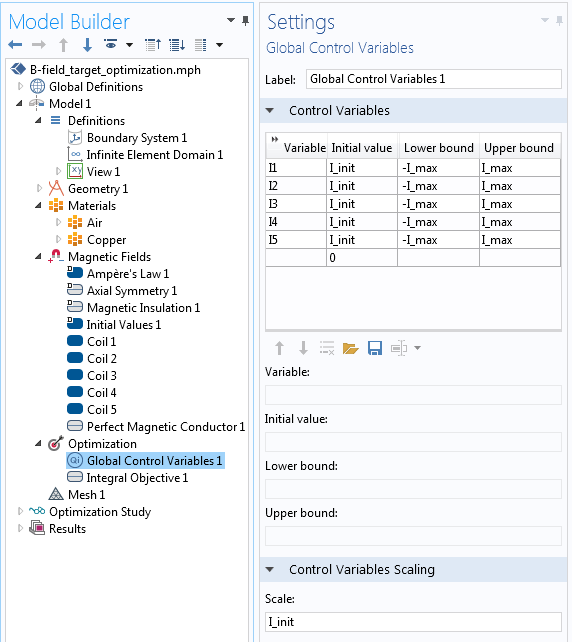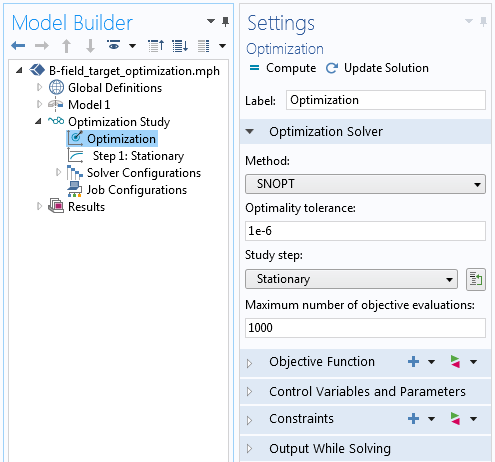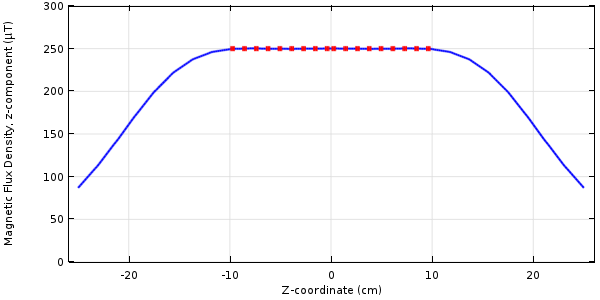### 通过场约束最小化功率

\begin{aligned}
& \underset{I_1, \ldots ,I_5}{\text{minimize:}}
& & \frac{1}{P_o}\sum_{k=1}^{5} P_{coil}^k \\
& \text{subject to:}
& & -I_{max} \leq I_1, \ldots ,I_5 \leq I_{max}\\
& & & 1 \le B_z^i/B_{0}, i=1, \ldots, M
\end{aligned}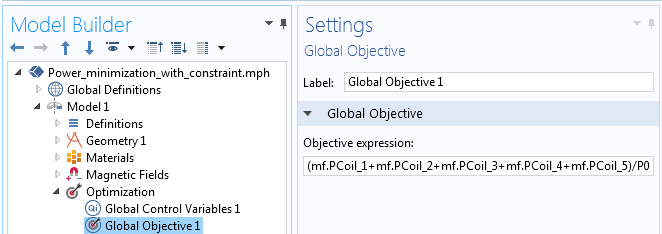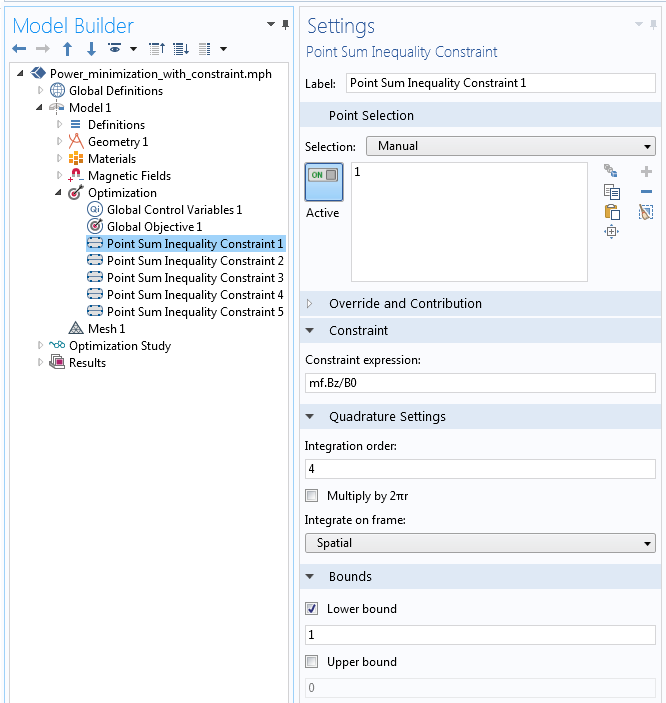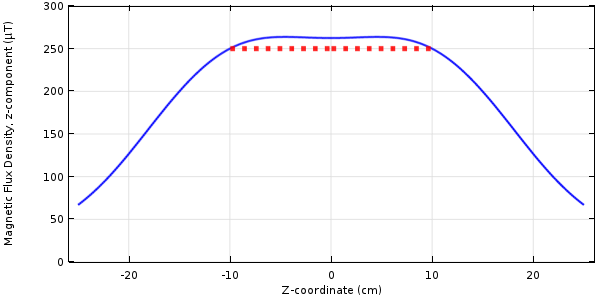### 通过场约束最小化梯度

\begin{aligned}
& \underset{I_1, \ldots ,I_5}{\text{minimize:}}
& & \frac{1}{L_0 B_{0}} \int_0^{L_0} \left( \frac{\partial B_z}{\partial z } \right) ^2 d l \\
& \text{subject to:}
& & -I_{max} \leq I_1, \ldots ,I_5 \leq I_{max}\\
& & & B_z(r=0,z=0) = B_0 \end{aligned}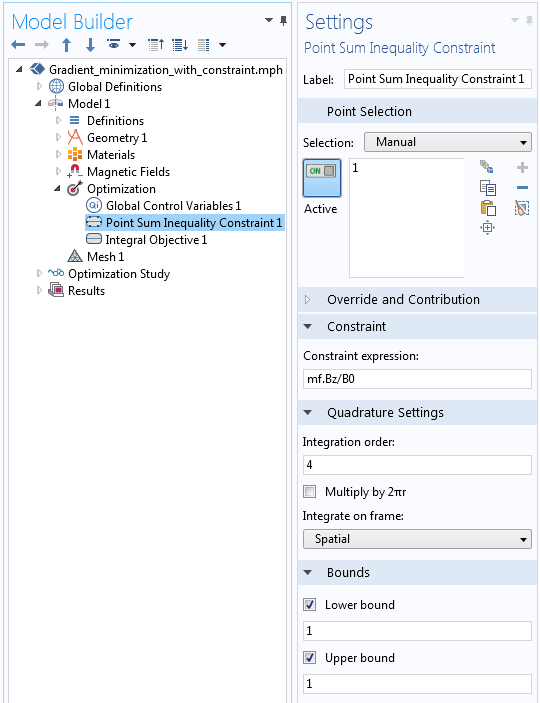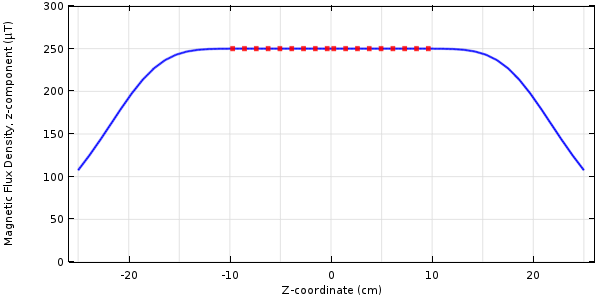SNOPT 优化求解器使用一种基于梯度的方法，并针对线圈电流的不同初始条件接近不同的局部最小值。虽然基于梯度的求解器会收敛到局部最小值，但不能保证这实际上是全局最小值。一般来说（除非我们对设计空间进行彻底的搜索），永远不可能保证优化的解是全局最小值。

### 更多资源

• 浏览 COMSOL 博客，了解有关电磁线圈建模的更多信息：